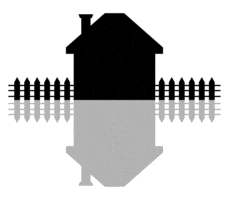# Math Worksheets Land

Math Worksheets For All Ages

# Math Worksheets Land

Math Worksheets For All Ages

Home > Grade Levels > High School Geometry >

# Geometric Transformations within a Plane Worksheets

In geometry we often have track or attempt to predict the movement of figures across a plane. This movement is often described as a geometric transformation. There are many different vocabulary terms that used to describe these changes in position. In this section of our site, we explain all this new language to you as well as how to best communicate this to others using common geometric terminology. While learning this language does not seem paramount, at this point, you will find this invaluable when you make your way to geometric proofs. You will learn how not only to track the position changes of all different types of figures, but how use formal language to explain this.

### Aligned Standard: High School Geometry - HSG-CO.A.2

• Answer Keys - These are for all the unlocked materials above.

### Homework Sheets

You determine the coordinates of shapes after they have undergone a translation of some sort.

• Homework 1 - A translation slides a figure to a different location. Move point A (-4, 1) right 4 units and down 1 unit. The new point A' is located at coordinates (0, 0).
• Homework 2 - Rotate point K (-6, 2) 90° counterclockwise around the origin. The point will move from Quadrant II to Quadrant III. The new point K' is located at (-2,-6).
• Homework 3 - Start with the point A (6, -6). Move the point 4 units left.

### Practice Worksheets

I find that most kids have trouble understanding how objects translate up and to the left. Here you will find a bunch of practice questions on that specific topic.

• Practice 1 - Graph the image of D (6, 8) after a translation 7 units down.
• Practice 2 - Graph the image of rectangle PQR after a rotation 90° clockwise around the origin.
• Practice 3 - Write the coordinates of the vertices after a translation 3 units left and 3 units up.

### Math Skill Quizzes

Filled with rotations and reflections across the axis.

• Quiz 1 - Examples of problems: Reflect over the y-axis. Translate right 4 units.
• Quiz 2 - Translate 4 units to left. These all about pushing shapes around.
• Quiz 3 - Rotate clockwise 180 degrees around (0,0).

### What are Geometric Transformations within a Plane?The word transformation just means to change. In the case of a geometric shape, it can mean changing in many different ways. The shape can change position, orientation, or it can even change shape’ in the case of folding. When we are describing this change, we often cite the points of the shape and describe where they start and where they end up. A transformation on a plane is a function that maps points on a particular plane to other point or coordinates in the plane. In other words, a function that takes one point and moves it to another location.

When doing transformation on a plane, we start by representing the image on that plane. For example, circles, lines, and curves, or any other type of figure. In this process, the image that exists before the transformation is called the pre-image, and the final picture is called the image. For example, if we have a function f [{x, y}]: = {x + 1, y +1 }. Then once the movement is complete, the image would have a radius 1 centered on (1, 1). This series of worksheets and lessons help students understand and classify the change of position of geometric shapes.

There are four commonly described types of transformation. Translations occur when a figure changes position in any direction. A reflection occurs when a figure crosses over either axis of a coordinate plane. This movement produces a mirror image (hence the name). A rotation occurs when a figure turns around a fixed point and changes the orientation. They can go in a clockwise or counterclockwise direction. If the figure changes in size by enlarging or reducing, we call this a dilation.

### Why Is It Important to Describe the Movement of Shapes and Objects?

It is often important to take a second and step back and evaluate why you are learning something. When it comes to learning to communicate a movement of something to another person, it is a vital skill. Regardless of the environment you are considering, being able to understand a location is key. Think about how integrated into our lives the GPS (Global Positioning System) is. We can barely travel around the block with out our mobile phones guiding our every step. GPS is merely an application of the type of math we are learning on the topic. While we really broke this concept down to the most primitive state, it is all not possible at all without addressing that fundamental concept. When you think about it, the applications of this foundation are limitless. It applies to anything that we are describing the movement of. The concept of the big three movements (rotations, reflections, and simple translations) are found in countless applications. All thanks to this basic concept.

Unlock all the answers, worksheets, homework, tests and more!
Save Tons of Time! Make My Life Easier Now

## Thanks and Don't Forget To Tell Your Friends!

I would appreciate everyone letting me know if you find any errors. I'm getting a little older these days and my eyes are going. Please contact me, to let me know. I'll fix it ASAP.